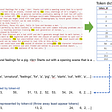# How to add function (Get F1-score) in Keras metrics and record F1 value after each epoch?

`from keras.callbacks import Callback,ModelCheckpointfrom keras.models import Sequential,load_modelfrom keras.layers import Dense, Dropoutfrom keras.wrappers.scikit_learn import KerasClassifierimport keras.backend as K`
`def get_f1(y_true, y_pred): #taken from old keras source code    true_positives = K.sum(K.round(K.clip(y_true * y_pred, 0, 1)))    possible_positives = K.sum(K.round(K.clip(y_true, 0, 1)))    predicted_positives = K.sum(K.round(K.clip(y_pred, 0, 1)))    precision = true_positives / (predicted_positives + K.epsilon())    recall = true_positives / (possible_positives + K.epsilon())    f1_val = 2*(precision*recall)/(precision+recall+K.epsilon())    return f1_val`
`## Define Modelinput_shape = X_train_tfidf.shapedef mlp_v2():        mdl = Sequential()    mdl.add(Dense(512, init='glorot_uniform', activation='relu',input_shape=(input_shape,)))    mdl.add(Dropout(0.5))    mdl.add(Dense(128, init='glorot_uniform', activation='relu'))    mdl.add(Dropout(0.5))    mdl.add(Dense(1, activation='sigmoid'))    mdl.compile(loss='binary_crossentropy', optimizer='adadelta', metrics=[get_f1])    mdl.summary()    return mdlmode_path = '../models/mlp_v2.h5'callbacks = [ModelCheckpoint(filepath=mode_path, save_best_only=True)]`
`## Run Modelestimator = KerasClassifier(build_fn=mlp_v2, epochs=5, batch_size=128)history = estimator.fit(X_train_tfidf.toarray(), y_train,\                        validation_data=(X_val_tfidf.toarray(),y_val),callbacks=callbacks)`

--

--

--

## More from Aakash Goel

Senior Data Scientist @ Fractal Analytics

Love podcasts or audiobooks? Learn on the go with our new app.

## “Generative Adversarial Networks” Science-Research, January 2022, Week 4 — summary from Arxiv…## Sequence-2-Sequence Model with Attention## Interesting Tensor Operations with Pytorch## Deep Learning — Beginners guide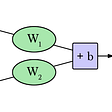## [Bayesian DL] 4. Types of Uncertainty## Exploring Defenses Against Adversarial Examples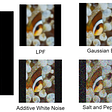## A line-by-line implementation guide to Word2Vec using Numpy## Machine learning is a zero-sum game!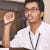## Aakash Goel

Senior Data Scientist @ Fractal Analytics

## Personal Protective Equipment (PPE) Detection Using Deep Learning Techniques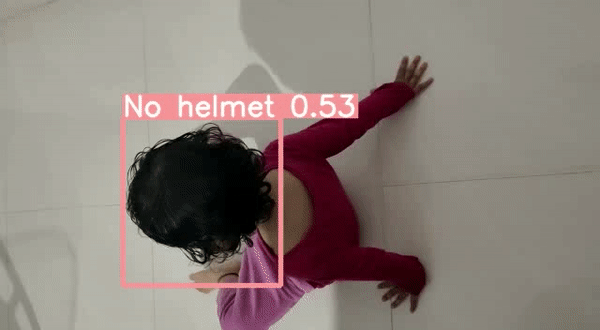## Digit Recognition: A Convolutional Neural Networks (CNN) Approach## The Simplest Neural Network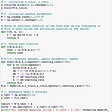## Image Caption Generation: Self-Attention